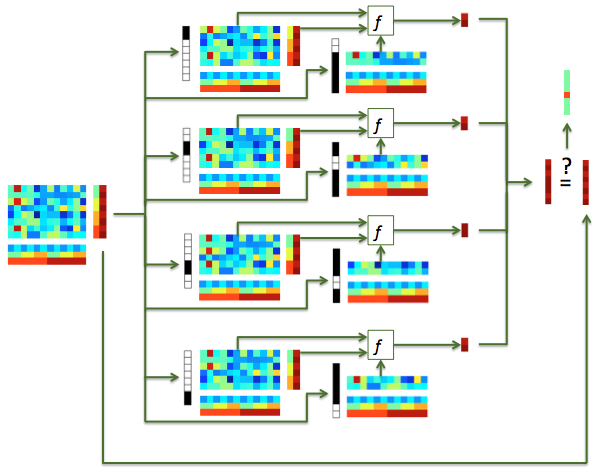# Classification analysis with cross-validation¶

## Background¶

In the Classification analysis exercise, data was split in even and odd runs, and a classifier trained and tested on these (respectively). In this exercise the use of cross-validation is shown using a dataset with N=10 chunks.

• data in a single chunk (here, the first run) is used as the test set

• all other data is used for the train set.

• the previous two steps are repeated for each of the N=10 chunks:

• for the i-th repetition, the i-th chunk is used for testing after training on all other chunks

• this gives a prediction for each sample in the dataset

• classification accuracy is, as before, computed by dividing the number of correct predictions by the total number of predictions

This procedure can be illustrated as follows:Illustration of classification with cross-validation. An input dataset (left), with 4 chunks and 2 targets in each chunk, is used for 4-fold cross-validation. In the i-th fold (i has values 1, 2, 3, 4), samples in the i-th chunk is used for testing, and the remaining samples used for training. Predictions are computed for each sample in each test set, and the results combined, yielding a prediction for each sample in the input dataset.

Compared to odd-even classification demonstrated earlier:

• for every classification step there is a larger training set, which generally means better signal-to-noise, leading to better estimates of the training parameters, and thus better classification.

• because a prediction is obtained for each sample in the dataset, more predictions are used to estimate classification accuracy, which leads to a better estimate of the true pattern discrimination.

## Single subject, n-fold cross-validation classification¶

For this exercise, load a dataset using subject s01’s T-statistics for every run (‘glm_T_stats_perrun.nii’) and the VT mask.

### Part 1: implement n-fold crossvalidation¶

In this part you have to do cross-validation manually.

• Implement n-fold crossvalidation as described above, using the LDA classifier.

• Compute classification accuracy.

• Show a confusion matrix.

### Part 2: use a partitioner¶

Because cross-validation is commonly used, CoSMoMVPA provides functions that define which samples are used for training and testing in each fold. Here, you can use cosmo nfold partitioner to obtain partitions for n-fold partitioning.

Notes:

Template: run nfold crossvalidate skl